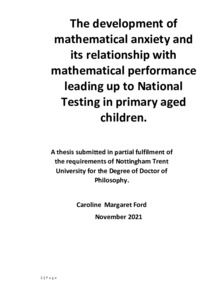# The development of mathematical anxiety and its relationship with mathematical performance leading up to National Testing in primary aged children

Ford, C.M., 2021. The development of mathematical anxiety and its relationship with mathematical performance leading up to National Testing in primary aged children. PhD, Nottingham Trent University.Preview
Text
Thesis Caroline Ford 2021 .pdf - Published version

## Abstract

The focus of this thesis is the development of mathematical anxiety and its relationship with mathematical performance. In particular, this relationship is explored with primary aged children during the year of National Testing. Mathematical anxiety is described as feelings of apprehension around undertaking mathematical tasks both in education and everyday life. Mathematical anxiety has been negatively associated with many different types of mathematical performance in both children and adults. In exploring this relationship account is taken of mathematical anxiety theory and cognitive (Working Memory, Non-verbal Intelligence and Reading ability) and emotional (Interest in mathematics, Trait and State Anxiety) factors. Employing a longitudinal design, the research explored the way in which the relationship between mathematical anxiety and mathematical performance changed at the different time points for the two groups of children.

A first study explored mathematical anxiety and three forms of mathematical performance, fluency, arithmetic, and word problem solving a year before the children undertook their National Standard Attainment Tests (SATs). Further studies explored mathematical anxiety and mathematical performance at the beginning of the year, just before and the end of the year in which the children completed their SATs.

There was a negative correlation between mathematical anxiety and mathematical performance, and this became stronger as children neared completion of their SATs. In the case of the older group the negative correlation continued after completion of the SATs. Evidence was found of a directional relationship between mathematical anxiety and mathematical performance. This directional relationship was from prior mathematical performance (Mathematical fluency and word problem solving) leading to higher levels of mathematical anxiety. Evidence was also found that interest in mathematics was a mediator in the relationship between mathematical anxiety and mathematical performance. This research emphasizes that the relationship between mathematical anxiety and mathematical performance is an important factor to be considered in primary education.

Item Type: Thesis Ford, C.M. November 2021 This work is the intellectual property of the author, Caroline Ford, you may copy up to 5% of this work for private study, or personal, non-commercial research. Any reuse of the information contained within this document should be fully reference quoting the author, title, university, degree level and pagination. Queries or requests for any use, or if a more substantial copy is required should be directed in the owner of the intellectual property rights. Schools > School of Social Sciences Laura Ward 17 May 2022 08:39 17 May 2022 08:39 https://irep.ntu.ac.uk/id/eprint/46329Edit View